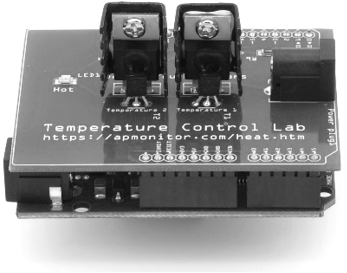## Dynamic Simulation in PythonA step response is a common evaluation of the dynamics of a simulated system. A linear time invariant (LTI) system can be described equivalently as a transfer function, a state space model, or solved numerically with and ODE integrator. This tutorial shows how to simulate a first and second order system in Python.Simulate Dynamics NotebookJupyter Notebook in Google Colab

#### First Order System Simulation

Consider a first order differential equation with constants K_p=3 and \tau_p=2, input u, and output response y.

$$\tau_p \frac{dy}{dt} = -y + K_p u$$

Three methods to represent this differential equation is as a (1) transfer function, (2) state space model, and (3) semi-explicit differential equation. Source code is included below to simulate a step response in these three forms.

#### 1. Transfer Function

$$\frac{Y(s)}{U(s)} = \frac{K_p}{\tau_p \,s + 1}$$

#### 2. State Space Model

$$\dot x = A x + B u$$

$$y = C x + D u$$

$$A = -\frac{1}{\tau_p} \quad B = \frac{K_p}{\tau_p} \quad C=1 \quad D=0$$

#### 3. Differential Equation

$$\frac{dy}{dt} = -\frac{1}{\tau_p} y + \frac{K_p}{\tau_p} u$$import numpy as np
from scipy import signal
import matplotlib.pyplot as plt
from scipy.integrate import odeint

# Simulate taup * dy/dt = -y + K*u
Kp = 3.0
taup = 2.0

# (1) Transfer Function
num = [Kp]
den = [taup,1]
sys1 = signal.TransferFunction(num,den)
t1,y1 = signal.step(sys1)

# (2) State Space
A = -1.0/taup
B = Kp/taup
C = 1.0
D = 0.0
sys2 = signal.StateSpace(A,B,C,D)
t2,y2 = signal.step(sys2)

# (3) ODE Integrator
def model3(y,t):
u = 1
return (-y + Kp * u)/taup
t3 = np.linspace(0,14,100)
y3 = odeint(model3,0,t3)

plt.figure(1)
plt.plot(t1,y1,'b--',linewidth=3,label='Transfer Fcn')
plt.plot(t2,y2,'g:',linewidth=2,label='State Space')
plt.plot(t3,y3,'r-',linewidth=1,label='ODE Integrator')
plt.xlabel('Time')
plt.ylabel('Response (y)')
plt.legend(loc='best')
plt.show()

#### Second Order System Simulation

##### 1. Laplace Domain, Transfer Function

$$\frac{Y(s)}{U(s)} = \frac{K_p}{\tau_s^2 s^2 + 2 \zeta \tau_s s + 1}e^{-\theta_p s}$$

##### 2. State Space Form

$$\begin{bmatrix}\dot x_1\\\dot x_2\end{bmatrix} = \begin{bmatrix}0&1\\-\frac{1}{\tau_s^2}&-\frac{2 \zeta}{\tau_s}\end{bmatrix} \begin{bmatrix}x_1\\x_2\end{bmatrix} + \begin{bmatrix}0\\\frac{K_p}{\tau_{s}^2}\end{bmatrix} u\left(t-\theta_p\right)$$

$$y = \begin{bmatrix}1 & 0\end{bmatrix} \begin{bmatrix}x_1\\x_2\end{bmatrix} + \begin{bmatrix}0\end{bmatrix} u$$

##### 3. Second Order Differential Equation

$$\tau_s^2 \frac{d^2y}{dt^2} + 2 \zeta \tau_s \frac{dy}{dt} + y = K_p \, u\left(t-\theta_p \right)$$Kp    = 2.0  # gain
tau   = 1.0  # time constant
zeta  = 0.25 # damping factor
theta = 0.0  # no time delay

import numpy as np
from scipy import signal
import matplotlib.pyplot as plt
from scipy.integrate import odeint

# tau * dy2/dt2 + 2*zeta*tau*dy/dt + y = Kp*u
Kp = 2.0    # gain
tau = 1.0   # time constant
zeta = 0.25 # damping factor
theta = 0.0 # no time delay
du = 1.0    # change in u

# (1) Transfer Function
num = [Kp]
den = [tau**2,2*zeta*tau,1]
sys1 = signal.TransferFunction(num,den)
t1,y1 = signal.step(sys1)

# (2) State Space
A = [[0.0,1.0],[-1.0/tau**2,-2.0*zeta/tau]]
B = [[0.0],[Kp/tau**2]]
C = [1.0,0.0]
D = 0.0
sys2 = signal.StateSpace(A,B,C,D)
t2,y2 = signal.step(sys2)

# (3) ODE Integrator
def model3(x,t):
y = x
dydt = x
dy2dt2 = (-2.0*zeta*tau*dydt - y + Kp*du)/tau**2
return [dydt,dy2dt2]
t3 = np.linspace(0,25,100)
x3 = odeint(model3,[0,0],t3)
y3 = x3[:,0]

plt.figure(1)
plt.plot(t1,y1*du,'b--',linewidth=3,label='Transfer Fcn')
plt.plot(t2,y2*du,'g:',linewidth=2,label='State Space')
plt.plot(t3,y3,'r-',linewidth=1,label='ODE Integrator')
y_ss = Kp * du
plt.plot([0,max(t1)],[y_ss,y_ss],'k:')
plt.xlim([0,max(t1)])
plt.xlabel('Time')
plt.ylabel('Response (y)')
plt.legend(loc='best')
plt.savefig('2nd_order.png')
plt.show()

#### Higher Order Simulation

A simple higher order simulation is the combination of n first order equations. The value of the time constant is 10/n in this example. The first equation is a first order differential expression.

$$\tau \frac{dy_1}{dt} = -y_1 + 1$$

Additional equations are also first order differential expressions for i = 2, n.

$$\tau \frac{dy_i}{dt} = -y_i + y_{i-1}$$